# Flow meter sizing for orifice

## What is orifice?

Orifice plate produce a constriction in the flow channel which results in a differential pressure across the constrian. The differential pressure is measured to measure the flowrate.

## Flow rate:

The flow rate can be derived from the Bernoulli’s principle:

Where prefix number represents the location 1 as the upstream and 2 as the downstream. From continuity, the velocities can be replaced by cross-sectional areas of the flow and the volumetric flow rate Q,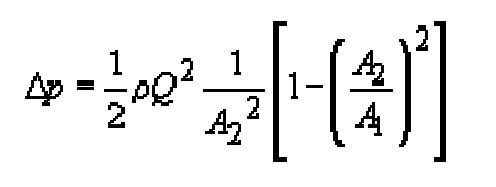Solving for the volumetric flowrate Q gives,Since the actual flow profile at location 2 downstream of the orifice is quite complex, thereby making the effective value of A2 uncertain, the following substitution introducing a flow coefficient Cf is made, and Cd is the discharge coefficient: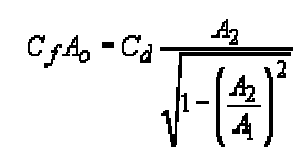where Ao is the area of the orifice. As a result, the volumetric flow rate Q for real flows is given by the equation,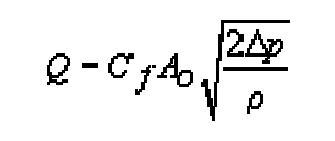The orifice plate can be made of any material, although stainless steel is the most common. The thickness of the plate used (1 / 8-1 / 2 ") is a function of line size, process temperature, pressure and differential pressure. The traditional hole is a thin circular plate (with a flange for handling) and for data), inserted into the pipeline between the two flanges of an orifice joint. This method of installation is cost-effective but requires closing the process whenever the plate is removed for maintenance or inspection. , an orifice connection allows the orifice to be removed from the process without depressurizing the line and closing the flow. In such accessories, the universal orifice plate, a circular plate with no tab, is used.

## Orifice Plate Sizing:

QM = Maximum flow in GPM Gb = Base S.G. [(S.G. of liquid @ 60°F (Water @ 60°F = 1)] N = 5.667 for GPM D = Pipe ID in inches GF = Flowing SG of liquid @ flowing temperature (see Crane A-6) hM = Meter differential in “WC S = Orifice ratio (reference Spink pg. 167 Table 12 for corresponding β)

Steam or Gas Orifice: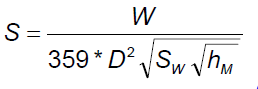basic steam equation: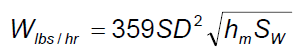Basic Equation Gas: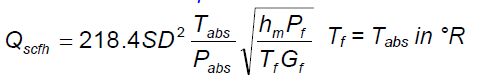SGgas=MW/29 W = Flow in lbs / hr SW = Specific Weight of vapor in lbs/ft3 = 1  Specific Volume For Steam, reference Crane A12 thru A18 (use 1/specific volume) For Gas, reference Crane A-8, column rho ‘ρ’) hM = Meter differential in “WC D = Pipe ID in inches S = Orifice ratio